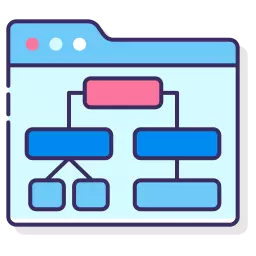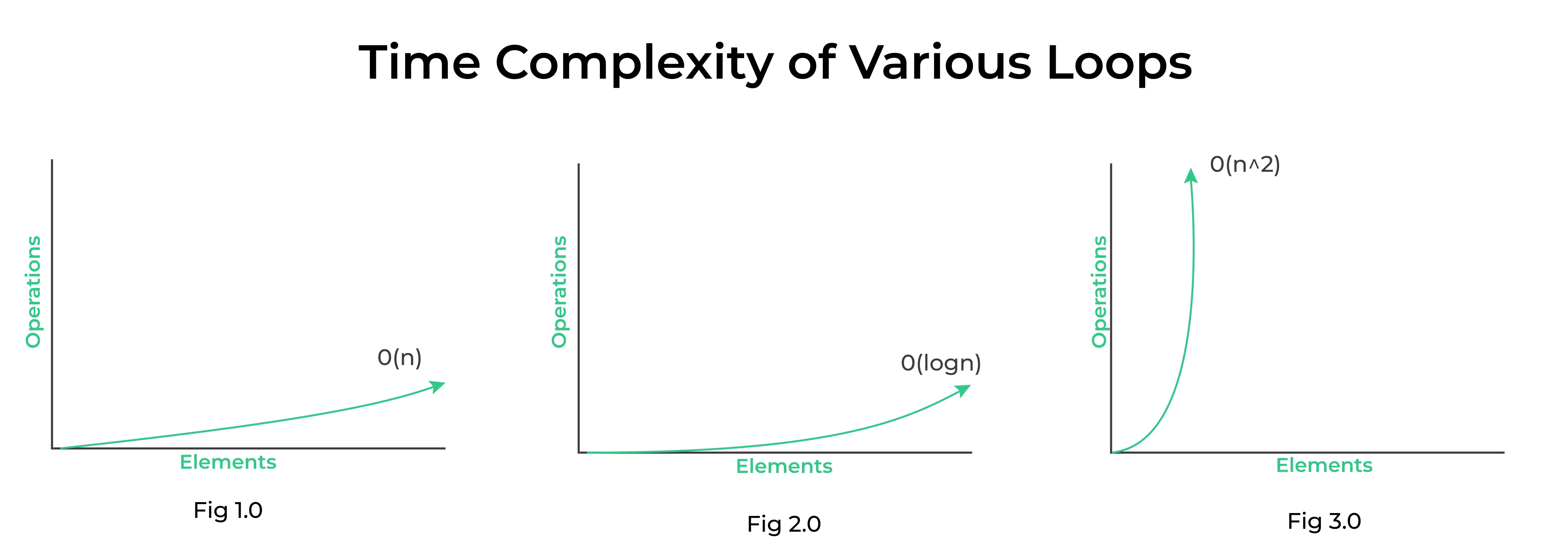# Time Complexity of Various Loops## Time Complexity of Various Loops

Time complexity is a measure of the computational resources an algorithm uses concerning the size of the input data.

It allows us to predict how the algorithm’s performance will scale as the input grows larger. In this article, you will get to learn about time complexity of various Loops.

## The Basics of Big O Notation:

Big O notation is a way to analyze and describe the efficiency or time complexity of algorithms in computer science. It helps us understand how an algorithm’s performance scales as the input size grows.

• Here are the basic points to know:
• implicity: Big O notation simplifies complex algorithms into their most significant terms. It focuses on the worst-case scenario.

• Common Notations:

• O(1): Constant time complexity. The algorithm’s runtime doesn’t depend on the input size.
• O(log n): Logarithmic time complexity. Common for efficient search algorithms like binary search.
• O(n): Linear time complexity. The runtime grows linearly with the input size.
• O(n^2): Quadratic time complexity. Common for nested loops.
• O(2^n): Exponential time complexity. Very inefficient and often impractical for large inputs.• Fig 1.0:  Time complexity of a simple loop when the loop variable is incremented or decremented by a constant amount.
• Fig 2.0: Time complexity of a loop when the loop variable is divided or multiplied by a constant amount.
• Fig 3.0: Time complexity of a nested loop.
Loop TypeTime Complexity (Big O Notation)Description
For LoopO(n)Iterates a fixed number of times (linear complexity).
While LoopO(n)Iterates until a certain condition is met (linear complexity).
Nested LoopO(n^k)k nested loops, each iterating n times (exponential complexity).
Nested Loops (with Break)O(n*m)k nested loops, each iterating n times, with early termination (quadratic complexity).
Do-While LoopO(n)Similar to a while loop, but guarantees at least one iteration (linear complexity).
RecursionVaries (e.g., O(2^n), O(n!))Depends on the recursive algorithm and branching factor.
Binary SearchO(log n)Reduces the search space by half in each iteration (logarithmic complexity).
For each LoopO(n)Iterates over all elements in a collection (linear complexity).

## Time complexity analysis of a single for and while loop

When a single `for` or `while` loop runs a constant number of times, its time complexity is expressed as O(1), which means it has a constant time complexity. This is because the number of iterations is not dependent on the size of the input or problem; it remains the same regardless of the input size. Here’s a brief explanation:

```for i in range(10):
print(i)
```
• In this for loop, it will always iterate 10 times, no matter the value of 10 or any other constant.
• Therefore, the time complexity of this loop is O(1), indicating that it takes a constant amount of time to execute, regardless of the input size.
• The same principle applies to a while loop with a constant number of iterations.

## Time complexity analysis of the nested for and while loops

Analyzing the time complexity of nested `for` and `while` loops involves considering the number of iterations in each loop and how they interact with one another. Here’s how you can analyze the time complexity of nested loops along with an example:

```n = 3  # Assuming n is the input size
for i in range(n):
for j in range(n):
print(i, j)
```

In this example, we have two nested `for` loops. Let’s break down the analysis:

• The outer loop iterates `n` times.
• For each iteration of the outer loop, the inner loop iterates `n` times.

To find the total number of iterations, we multiply the iterations of the outer loop by the iterations of the inner loop, which is `n * n = n^2`.

So, the total number of iterations is proportional to `n^2`, and the time complexity of these nested `for` loops is O(n^2).

## Steps to analyze the time complexity of the loop

Analyzing the time complexity of a loop can be broken down into several steps to make the process systematic. Here are six steps to analyze the time complexity of a loop:

1. Identify the Loop: First, identify the loop in your code that you want to analyze for time complexity. Ensure you have a clear understanding of what the loop is doing and how it’s structured.

2. Count the Iterations: Determine how many times the loop will iterate based on the input or the size of the problem. This may involve examining the loop’s control variable, conditions, and any exit points.

3. Analyze Operations Inside the Loop: Consider the operations performed inside the loop. Assign a constant time complexity to each operation. For example, basic arithmetic, assignments, and comparisons are usually considered O(1) or constant time. If there are nested loops or function calls, consider their time complexities as well.

4. Calculate the Total Operations: Multiply the number of iterations (step 2) by the time complexity of the operations inside the loop (step 3). This gives you the total number of basic operations executed by the loop.

5. Simplify and Express as Big O: Simplify the expression from step 4, if necessary, to obtain a simplified equation that describes the growth rate of the loop’s time complexity in terms of Big O notation. Remove any lower-order terms or constants, as they don’t affect the overall growth rate.

6. Determine the Final Time Complexity: The simplified expression obtained in step 5 represents the time complexity of the loop. Express it in Big O notation (e.g., O(n), O(n^2), O(log n)) to describe how the time complexity scales with the size of the input or problem.

##### Conclusion

In conclusion, grasping the time complexity of various loops empowers programmers to write more efficient and scalable code. By choosing the right loop structure for the task at hand, you can significantly impact the performance of your programs.

### Related Banners

Get PrepInsta Prime & get Access to all 200+ courses offered by PrepInsta in One Subscription

## Get over 200+ course One Subscription

Courses like AI/ML, Cloud Computing, Ethical Hacking, C, C++, Java, Python, DSA (All Languages), Competitive Coding (All Languages), TCS, Infosys, Wipro, Amazon, DBMS, SQL and others